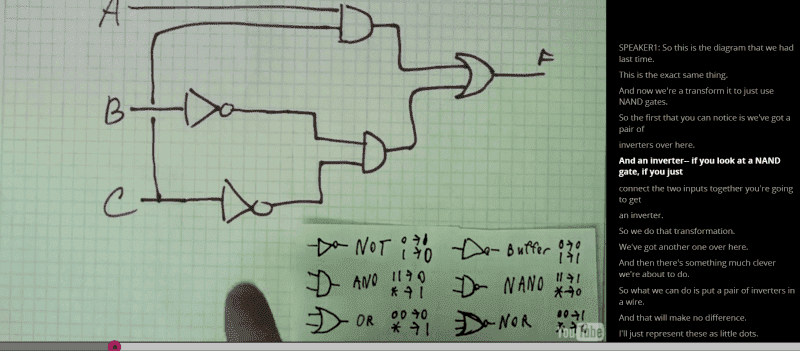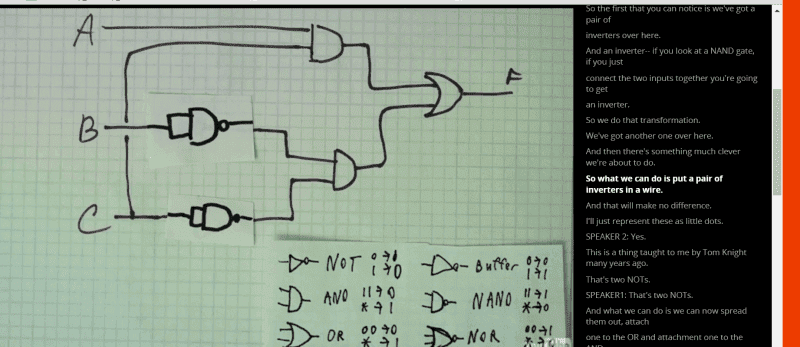# Converting logic diagram to only NAND gates

## Homework Statement

How is he able to just swap the inverter for a NAND gate? Doesn't a NAND gate require two things?

See the text on the right for his explanation
[/B]## The Attempt at a Solution

gneill
Mentor
How is he able to just swap the inverter for a NAND gate? Doesn't a NAND gate require two things?
What sort of "things" did you have in mind? If the inputs are tied together then the only inputs available to the NAND are both inputs "1" or both inputs "0". What does a NAND gate output for each case?

NascentOxygen
Staff Emeritus
How is he able to just swap the inverter for a NAND gate?
So, we are revisiting this topic! :) We thought you would learn about the NAND inverter from your previous thread, but I think you dropped out and didn't see it through to its conclusion.

You can join the two inputs together, which gives both inputs either 1 or both inputs 0, and the output becomes the NOT of this. So, yes, a NAND gate with a signal applied to both its inputs acts as a NOT gate.

CWatters
Homework Helper
Gold Member
Or look at it this way.. The NAND truth table is

00=1
01=1
10=1
11=0

if both inputs are wired together they are always the same, so the only combinations that can occur are..

00=1
11=0

There is another way to turn a NAND gate into an inverter - You could just connect one input to a logic 1 permanently....

10=1
11=0